# Lesson 6: Factoring Polynomials

16 Jul 2020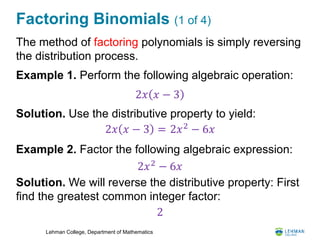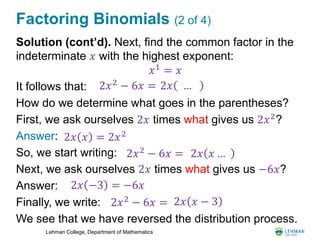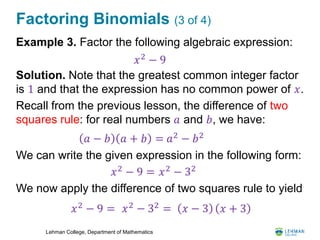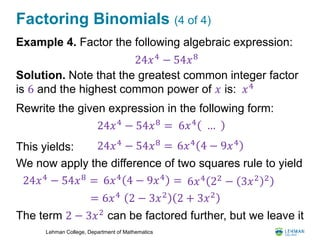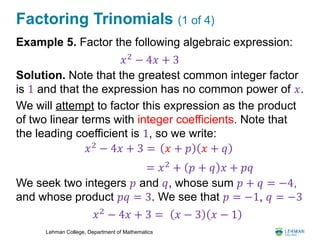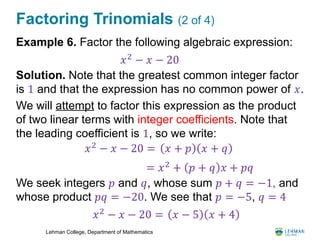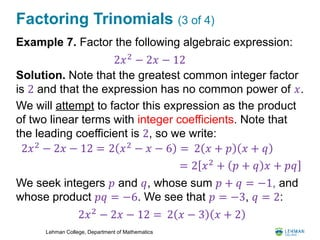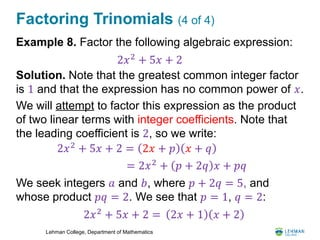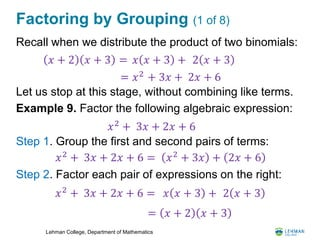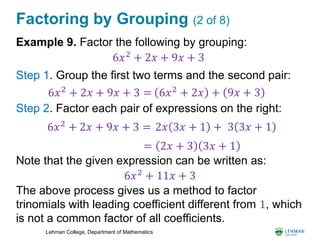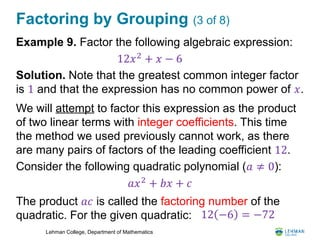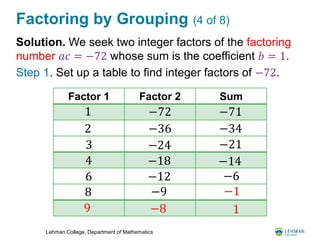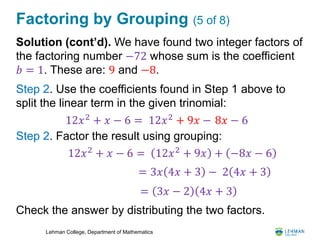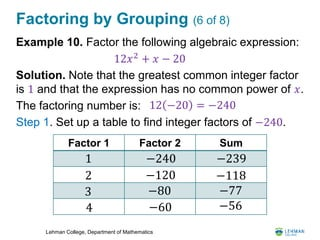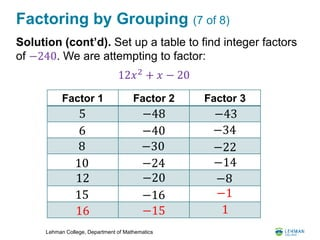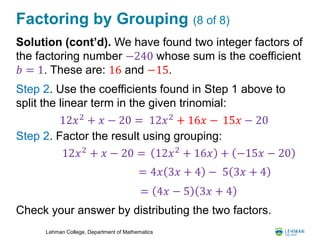1 sur 17

### Lesson 6: Factoring Polynomials

• 1. 𝐏𝐓𝐒 𝟑 Bridge to Calculus Workshop Summer 2020 Lesson 6 Factoring Polynomials "I have had my results for a long time: but I do not yet know how I am to arrive at them.“ - Carl Friedrich Gauss
• 2. Lehman College, Department of Mathematics Factoring Binomials (1 of 4) The method of factoring polynomials is simply reversing the distribution process. Example 1. Perform the following algebraic operation: Solution. Use the distributive property to yield: Example 2. Factor the following algebraic expression: Solution. We will reverse the distributive property: First find the greatest common integer factor: 2𝑥 𝑥 − 3 2𝑥 𝑥 − 3 = 2𝑥2 − 6𝑥 2𝑥2 − 6𝑥 2
• 3. Lehman College, Department of Mathematics Factoring Binomials (2 of 4) Solution (cont’d). Next, find the common factor in the indeterminate 𝑥 with the highest exponent: It follows that: How do we determine what goes in the parentheses? First, we ask ourselves 2𝑥 times what gives us 2𝑥2 ? Answer: So, we start writing: Next, we ask ourselves 2𝑥 times what gives us −6𝑥? Answer: Finally, we write: We see that we have reversed the distribution process. 𝑥1 = 𝑥 2𝑥2 − 6𝑥 = 2𝑥 … 2𝑥 𝑥 = 2𝑥2 2𝑥2 − 6𝑥 = 2𝑥 𝑥 … 2𝑥 −3 = −6𝑥 2𝑥2 − 6𝑥 = 2𝑥 𝑥 − 3
• 4. Lehman College, Department of Mathematics Factoring Binomials (3 of 4) Example 3. Factor the following algebraic expression: Solution. Note that the greatest common integer factor is 1 and that the expression has no common power of 𝑥. Recall from the previous lesson, the difference of two squares rule: for real numbers 𝑎 and 𝑏, we have: We can write the given expression in the following form: We now apply the difference of two squares rule to yield 𝑥2 − 9 𝑎 − 𝑏 𝑎 + 𝑏 = 𝑎2 − 𝑏2 𝑥2 − 9 = 𝑥2 − 32 𝑥2 − 9 = 𝑥2 − 32 = 𝑥 − 3 𝑥 + 3
• 5. Lehman College, Department of Mathematics Factoring Binomials (4 of 4) Example 4. Factor the following algebraic expression: Solution. Note that the greatest common integer factor is 6 and the highest common power of 𝑥 is: Rewrite the given expression in the following form: This yields: We now apply the difference of two squares rule to yield The term 2 − 3𝑥2 can be factored further, but we leave it 24𝑥4 − 54𝑥8 𝑥4 24𝑥4 − 54𝑥8 = 6𝑥4 … 24𝑥4 − 54𝑥8 = 6𝑥4 4 − 9𝑥4 24𝑥4 − 54𝑥8 = 6𝑥4 4 − 9𝑥4 = 6𝑥4 22 − 3𝑥2 2 = 6𝑥4 2 − 3𝑥2 2 + 3𝑥2
• 6. Lehman College, Department of Mathematics Factoring Trinomials (1 of 4) Example 5. Factor the following algebraic expression: Solution. Note that the greatest common integer factor is 1 and that the expression has no common power of 𝑥. We will attempt to factor this expression as the product of two linear terms with integer coefficients. Note that the leading coefficient is 1, so we write: We seek two integers 𝑝 and 𝑞, whose sum 𝑝 + 𝑞 = −4, and whose product 𝑝𝑞 = 3. We see that 𝑝 = −1, 𝑞 = −3 𝑥2 − 4𝑥 + 3 𝑥2 − 4𝑥 + 3 = 𝑥 + 𝑝 𝑥 + 𝑞 = 𝑥2 + 𝑝 + 𝑞 𝑥 + 𝑝𝑞 𝑥2 − 4𝑥 + 3 = 𝑥 − 3 𝑥 − 1
• 7. Lehman College, Department of Mathematics Factoring Trinomials (2 of 4) Example 6. Factor the following algebraic expression: Solution. Note that the greatest common integer factor is 1 and that the expression has no common power of 𝑥. We will attempt to factor this expression as the product of two linear terms with integer coefficients. Note that the leading coefficient is 1, so we write: We seek integers 𝑝 and 𝑞, whose sum 𝑝 + 𝑞 = −1, and whose product 𝑝𝑞 = −20. We see that 𝑝 = −5, 𝑞 = 4 𝑥2 − 𝑥 − 20 𝑥2 − 𝑥 − 20 = 𝑥 + 𝑝 𝑥 + 𝑞 = 𝑥2 + 𝑝 + 𝑞 𝑥 + 𝑝𝑞 𝑥2 − 𝑥 − 20 = 𝑥 − 5 𝑥 + 4
• 8. Lehman College, Department of Mathematics Factoring Trinomials (3 of 4) Example 7. Factor the following algebraic expression: Solution. Note that the greatest common integer factor is 2 and that the expression has no common power of 𝑥. We will attempt to factor this expression as the product of two linear terms with integer coefficients. Note that the leading coefficient is 2, so we write: We seek integers 𝑝 and 𝑞, whose sum 𝑝 + 𝑞 = −1, and whose product 𝑝𝑞 = −6. We see that 𝑝 = −3, 𝑞 = 2: 2𝑥2 − 2𝑥 − 12 2𝑥2 − 2𝑥 − 12 = 2 𝑥 + 𝑝 𝑥 + 𝑞 = 2 𝑥2 + 𝑝 + 𝑞 𝑥 + 𝑝𝑞 2𝑥2 − 2𝑥 − 12 = 2 𝑥 − 3 𝑥 + 2 2 𝑥2 − 𝑥 − 6 =
• 9. Lehman College, Department of Mathematics Factoring Trinomials (4 of 4) Example 8. Factor the following algebraic expression: Solution. Note that the greatest common integer factor is 1 and that the expression has no common power of 𝑥. We will attempt to factor this expression as the product of two linear terms with integer coefficients. Note that the leading coefficient is 2, so we write: We seek integers 𝑎 and 𝑏, where 𝑝 + 2𝑞 = 5, and whose product 𝑝𝑞 = 2. We see that 𝑝 = 1, 𝑞 = 2: 2𝑥2 + 5𝑥 + 2 2𝑥2 + 5𝑥 + 2 = 2𝑥 + 𝑝 𝑥 + 𝑞 = 2𝑥2 + 𝑝 + 2𝑞 𝑥 + 𝑝𝑞 2𝑥2 + 5𝑥 + 2 = 2𝑥 + 1 𝑥 + 2
• 10. Lehman College, Department of Mathematics Factoring by Grouping (1 of 8) Recall when we distribute the product of two binomials: Let us stop at this stage, without combining like terms. Example 9. Factor the following algebraic expression: Step 1. Group the first and second pairs of terms: Step 2. Factor each pair of expressions on the right: 𝑥 + 2 𝑥 + 3 = = 𝑥2 + 3𝑥 + 𝑥 𝑥 + 3 + 2 𝑥 + 3 2𝑥 + 6 𝑥2 + 3𝑥 + 2𝑥 + 6 𝑥2 + 3𝑥 + 2𝑥 + 6 = 𝑥2 + 3𝑥 + 2𝑥 + 6 𝑥2 + 3𝑥 + 2𝑥 + 6 = 𝑥 𝑥 + 3 + 2 𝑥 + 3 = 𝑥 + 2 𝑥 + 3
• 11. Lehman College, Department of Mathematics Factoring by Grouping (2 of 8) Example 9. Factor the following by grouping: Step 1. Group the first two terms and the second pair: Step 2. Factor each pair of expressions on the right: Note that the given expression can be written as: The above process gives us a method to factor trinomials with leading coefficient different from 1, which is not a common factor of all coefficients. 6𝑥2 + 2𝑥 + 9𝑥 + 3 6𝑥2 + 2𝑥 + 9𝑥 + 3 2𝑥 3𝑥 + 1 + 3 3𝑥 + 1 6𝑥2 + 2𝑥 + 9𝑥 + 3 = 6𝑥2 + 2𝑥 + 9𝑥 + 3 = = 2𝑥 + 3 3𝑥 + 1 6𝑥2 + 11𝑥 + 3
• 12. Lehman College, Department of Mathematics Factoring by Grouping (3 of 8) Example 9. Factor the following algebraic expression: Solution. Note that the greatest common integer factor is 1 and that the expression has no common power of 𝑥. We will attempt to factor this expression as the product of two linear terms with integer coefficients. This time the method we used previously cannot work, as there are many pairs of factors of the leading coefficient 12. Consider the following quadratic polynomial (𝑎 ≠ 0): The product 𝑎𝑐 is called the factoring number of the quadratic. For the given quadratic: 12𝑥2 + 𝑥 − 6 𝑎𝑥2 + 𝑏𝑥 + 𝑐 12 −6 = −72
• 13. Lehman College, Department of Mathematics Factoring by Grouping (4 of 8) Solution. We seek two integer factors of the factoring number 𝑎𝑐 = −72 whose sum is the coefficient 𝑏 = 1. Step 1. Set up a table to find integer factors of −72. Factor 1 Factor 2 Sum 1 −72 −71 2 −36 −34 3 −24 −21 4 −18 −14 6 −12 −6 8 −9 −1 9 −8 1
• 14. Lehman College, Department of Mathematics Factoring by Grouping (5 of 8) Solution (cont’d). We have found two integer factors of the factoring number −72 whose sum is the coefficient 𝑏 = 1. These are: 9 and −8. Step 2. Use the coefficients found in Step 1 above to split the linear term in the given trinomial: Step 2. Factor the result using grouping: Check the answer by distributing the two factors. 12𝑥2 + 𝑥 − 6 = 12𝑥2 + 9𝑥 − 8𝑥 − 6 12𝑥2 + 𝑥 − 6 = 12𝑥2 + 9𝑥 + −8𝑥 − 6 = 3𝑥 4𝑥 + 3 − 2 4𝑥 + 3 = 3𝑥 − 2 4𝑥 + 3
• 15. Lehman College, Department of Mathematics Factoring by Grouping (6 of 8) Example 10. Factor the following algebraic expression: Solution. Note that the greatest common integer factor is 1 and that the expression has no common power of 𝑥. The factoring number is: Step 1. Set up a table to find integer factors of −240. 12𝑥2 + 𝑥 − 20 12 −20 = −240 Factor 1 Factor 2 Sum 1 −240 −239 2 −120 −118 3 −80 −77 4 −60 −56
• 16. Lehman College, Department of Mathematics Factoring by Grouping (7 of 8) Solution (cont’d). Set up a table to find integer factors of −240. We are attempting to factor: 12𝑥2 + 𝑥 − 20 Factor 1 Factor 2 Factor 3 5 −48 −43 6 −40 −34 8 −30 −22 10 −24 −14 12 −20 −8 15 −16 −1 16 −15 1
• 17. Lehman College, Department of Mathematics Factoring by Grouping (8 of 8) Solution (cont’d). We have found two integer factors of the factoring number −240 whose sum is the coefficient 𝑏 = 1. These are: 16 and −15. Step 2. Use the coefficients found in Step 1 above to split the linear term in the given trinomial: Step 2. Factor the result using grouping: Check your answer by distributing the two factors. 12𝑥2 + 𝑥 − 20 = 12𝑥2 + 16𝑥 − 15𝑥 − 20 12𝑥2 + 𝑥 − 20 = 12𝑥2 + 16𝑥 + −15𝑥 − 20 = 4𝑥 3𝑥 + 4 − 5 3𝑥 + 4 = 4𝑥 − 5 3𝑥 + 4